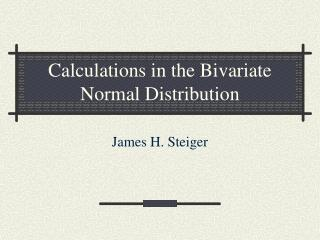DownloadDownload PresentationCalculations in the Bivariate Normal Distribution

# Calculations in the Bivariate Normal Distribution

Download Presentation## Calculations in the Bivariate Normal Distribution

- - - - - - - - - - - - - - - - - - - - - - - - - - - E N D - - - - - - - - - - - - - - - - - - - - - - - - - - -
##### Presentation Transcript

1. Calculations in the Bivariate Normal Distribution James H. Steiger

2. Problem 1 • Suppose that, for adult males, heights and weights have a distribution which is approximately bivariate normal. Heights have a mean of 70 and a standard deviation of 2.5. Weights have a mean of 150 and a standard deviation of 25. The correlation between height and weight is .60. • What percentage of men weigh more than 200?

3. Answer to problem 1 • 2.28%

4. Problem 2 • What percentage of men are less than 65 inches tall?

5. Answer to Problem 2 • 2.28%

6. Problem 3 • What percentage of men who are exactly 75 inches tall weigh less than 170 pounds?

7. Answer to Problem 3 • 30.85%

8. Problem 4 • What percentage of men who weigh 210 pounds are less than 70 inches tall?

9. Answer to Problem 4 • 3.59%

10. Problem 5 • Suppose you are 78 inches tall (and male). If you weigh 230 pounds, what is the percentile rank of your weight among people the same height as you?

11. Answer to Problem 5 • 94.52